## Fermi Dirac Function in Semiconductor:

It is important to know what energies are possessed by a mobile carrier in a solid or semiconductor. This relationship is referred to as energy distribution function. We are concerned with the distribution of energy of the free electrons in a metal. The Fermi Dirac Function statistics enables us to find the number of free electrons dnE per unit volume, within energy range E to E + dE at temperature T.

The number of free electrons per cubic metre of a metal whose energies lie in the interval E to E + dE is given by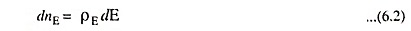where dnE represents the number of free electrons per cubic metre whose energies lie in the energy interval dE, and ρE represents the density of electrons in the energy interval dE.

In case of free electrons, it can be safely assumed that there is no potential variation within the metal. Accordingly, each cubic metre of the metal must have the same number of electrons. That is, the density in space (electrons/m3) is a constant. However, within each unit volume of metal there will be electrons having all possible energies. It is this distribution in energy that is represented by ρE. ρE represents the number of electrons per eV per m3 of the metal. The function ρE may be expressed as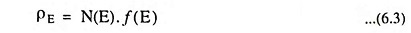where N(E) is the density of states (number of states per eV per cubic metre) in the conduction band, and f(E) is the probability that a quantum state with energy E is occupied by an electron.

The density of states, N(E) is found to be proportional to the half power of energy i.e.,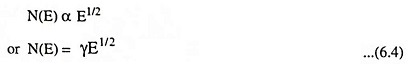where γ is a proportionality constant and is defined as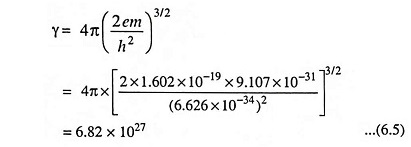where m is the mass of electron in kg, and h is Planck’s constant in joule-seconds.

The dimensions of γ are (m-3) (eV)-3/2.

The equation for f(E) is known as Fermi Dirac probability function, and specifies the fraction of all states at energy E (electron volts) occupied under conditions of thermal equilibrium.

According to the principle of quantum mechanics, the Fermi Dirac probability function is given by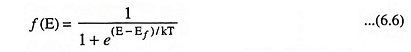where k is Boltzmann’s constant (k = 8.62 x 10-5 eV/K). The function f(E), the Fermi Dirac distribution function, gives the probability that an available energy state at E will be occupied by an electron at absolute temperature T. The quantity EF is called the Fermi level, and it represents an important quantity in the analysis of semiconductor behavior. We notice that, for an energy E equal to the Fermi level energy EF, the occupation probability is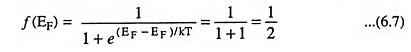Thus an energy state at the Fermi level has a probability of 50 per cent of being occupied by an electron.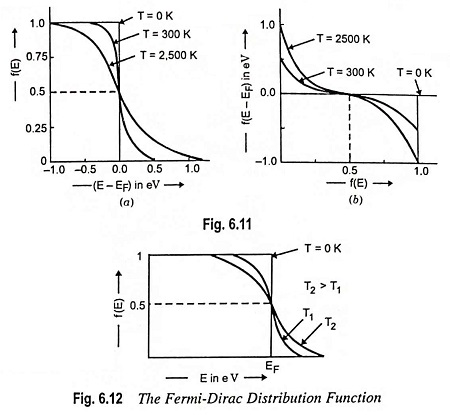A plot of f(E) versus E – EF is depicted in Fig. 6.11 (a) and of E – EF versus f(E) in Fig. 6.11 (b), both for T = 0 K, and for larger values of temperature.

A closer examination of f(E) indicates that at 0 K the distribution takes the simple rectangular form depicted in Fig. 6.12. With T = 0 in the denominator of the exponent, f(E) is 1/1+0 = 1 when the exponent is negative (E < EF), and is 1/1+∞ = 0 when the exponent is positive (E > EF). This rectangular distribution implies that at 0 K every available energy state up to EF is filled up with electrons, and all states above EF are empty.

From Eqs. (6.3), (6.4) and (6.6), we have at absolute zero temperature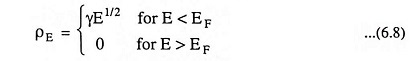At temperatures higher than 0 K, some probability exists for states above the Fermi level to be filled. For ex­ample, at T = T1 in Fig. 6.12, there is some prob­ability f(E) that states above EF are filled, and there is a corresponding probability [1 – f(E)] that states below EF are empty. The Fermi Dirac function is sym­metrical about EF for all temperatures; that is, the probability f(EF + ΔE) that a state ΔE above EF is filled is the same as the probability [1 – f(EF + ΔE)] that a state ΔE below EF is empty. The symmetry of the distribution of empty and filled states about EF makes the Fermi level a natural reference point in calculations of electron and hole concentrations in semiconduc­tors.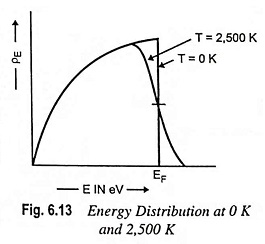The Fermi Level: An expression for EF can be had from energy distribution curve depicted in Fig. 6.13 at 0 K. The area under the curve represents the total number of free electrons (per cubic metre of metal). Thus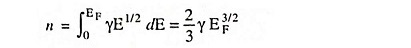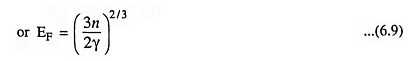Substituting γ = 6.82 x 1027 from Eq. (6.5), we have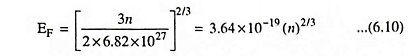The density n varies from metal to metal, therefore, EF will vary from metal to metal. The density of electrons n and so EF may be determined by knowing the specific gravity, the atomic weight, and the number of free electrons per atom. For most of the metals, the numerical value of EF is less than 10 eV.

Scroll to Top